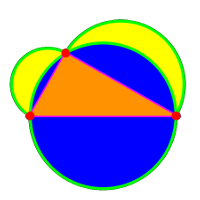Math E-320:
Teaching Math with a Historical Perspective
Mathematics E-320:
Instructor: Oliver Knill
Office: SciCtr 434

This course has a final project rather than a final exam. Here is some information about the expected form an format. The title of the project is

## The 10 greatest moments in mathematics

The minimal requirement is a list of 10 topics starting with the best, then the second best etc. Each choice should have an explanation, the time of the moment and maybe mathematicians involved. There is no length requirement. You are free to expand any of the topics into a larger framework. Each of the 10 moments could have the following form. The great moment can be a result, an object a concept or a bridge between different subjects. Below are four examples (not always very serious). You can extend any of the subjects to a longer paper, if you like. It is not required however. It is important to give ALL references and source you have consulted. Do not copy paste sources except for citation reasons, where you clearly mark the beginning and the end of the citation.

#### Great moment Nr 1: The hairy ball theorem

The hairy ball theorem states that however one combs a hairy ball, there always will be a cowlick. It is an important result because it is prototype of a theorem in algebraic topology, and a result which can be explained to the "man on the street". The result is a consequence of the fact that the sphere has Euler characteristic 2 and that this is the sum of the degrees of equilibrium points of a vector field. Without a cowlick, we would have Euler characteristic zero. The hairy ball theorem should not be confused with the "no hair theorem" in general relativity.

Sources:
1. Wikipedia article on Hairy Ball Theorem. Accessed, March 8, 2010

#### Great moment Nr 2: The emergence of number 4

The biggest moment of mathematics is definitely the appearance of the number 4. The reason is that the number 4 is the smallest number which is not a prime nor a unit. The number 4 is very special because 22 = 2*2 = 2+2. It is the only number which solves the system of Diophantine equations
```xx = x*x = x+x.
```
An other reason is that without the number 4, the number system would be incomplete. It would be terrible to count 1,2,3,5. The number 4 is also the smallest even number which is the sum of two primes. It is also the smallest number which is between two prime twins and the smallest composite number that is equal to the sum of its prime factors.

Sources:
1. Conway and Guy: The book of Numbers.
2. Wikipedia: http://en.wikipedia.org/wiki/4_(number) accessed, March 8, 2010

#### Great moment Nr 3: The "tertium non datur"

The concept of "tertium non datur" is the "law of excluded middle". It is an important argument principle which allows for example to establish that there are irrational numbers a,b, such that ab is rational. The first possibility is that sqrt(2)sqrt(2) is rational. In that case, we have a solution. A second possibility is that c = sqrt(2)sqrt(2) is irrational. But then, csqrt(2) = 2 is rational. There is no third possibility. One has to appreciate the argument, because in daily life, there is not such a thing. It is not that either "the cat is cute" or "the cat is not cute". There is a whole spectrum of possibilities from "the cat is awfully ugly" over "the cat is ok: its just a cat" to "the cat is so super cute and sweet". There is a branch of mathematical logic, the so called "fuzzy logic", which allows to capture these nuances of cats. Well, cats are fuzzy creatures anyway.

Sources:
1. Wikipedia http://en.wikipedia.org/wiki/Law_of_excluded_middle accessed, March 8, 2010

#### Great moment Nr 4: Bridging Math with Sex

The first mathematical study of population growth has been done by Fibonnacci (named after Leonardo Bonacci, Leonardo Fibonacci). It models the reproduction of rabbits. At time n, there are a(n) rabbits. Since both the a(n-1) rabbits from the previous year and the a(n) from the current year can reproduce, there are a(n) + a(n-1) rabbits next year. Starting wit 1,1 tis rule leads to the numbers
```   1,1,2,3,5,8,13,...
```
The Fibonacci sequence bridges the concept of difference equations with the biological problem of exponential population growth. An other place where mathematics bridges the concepts is the optimal strategy for finding a partner. How many people does one have to date to get the optimal chance to find the right one? If one marries the first one, then one has not have any comparisons and could have missed a much better one. When waiting too long however, the bar has been rising too much and one always compares with the best of the previous samples. One will never end up with a partner any more. (This example is from [Cladwell 2003]). An other example in the book of Cladwell is the oscillatory model of love. The harmonic oscillator can explain some up and downs in relationships, like the famous Brangelina cycle.

Sources
1. Clio Cladwell. Mathematics and Sex, Allen and Unwin, 2003.
2. The natural Enquirer: Angelina and Brad Split: June 3, 2009.
3. Entertainment Daily: Brangelina split rumors spark `Brad-Jen' reunion speculation, January 26, 2010